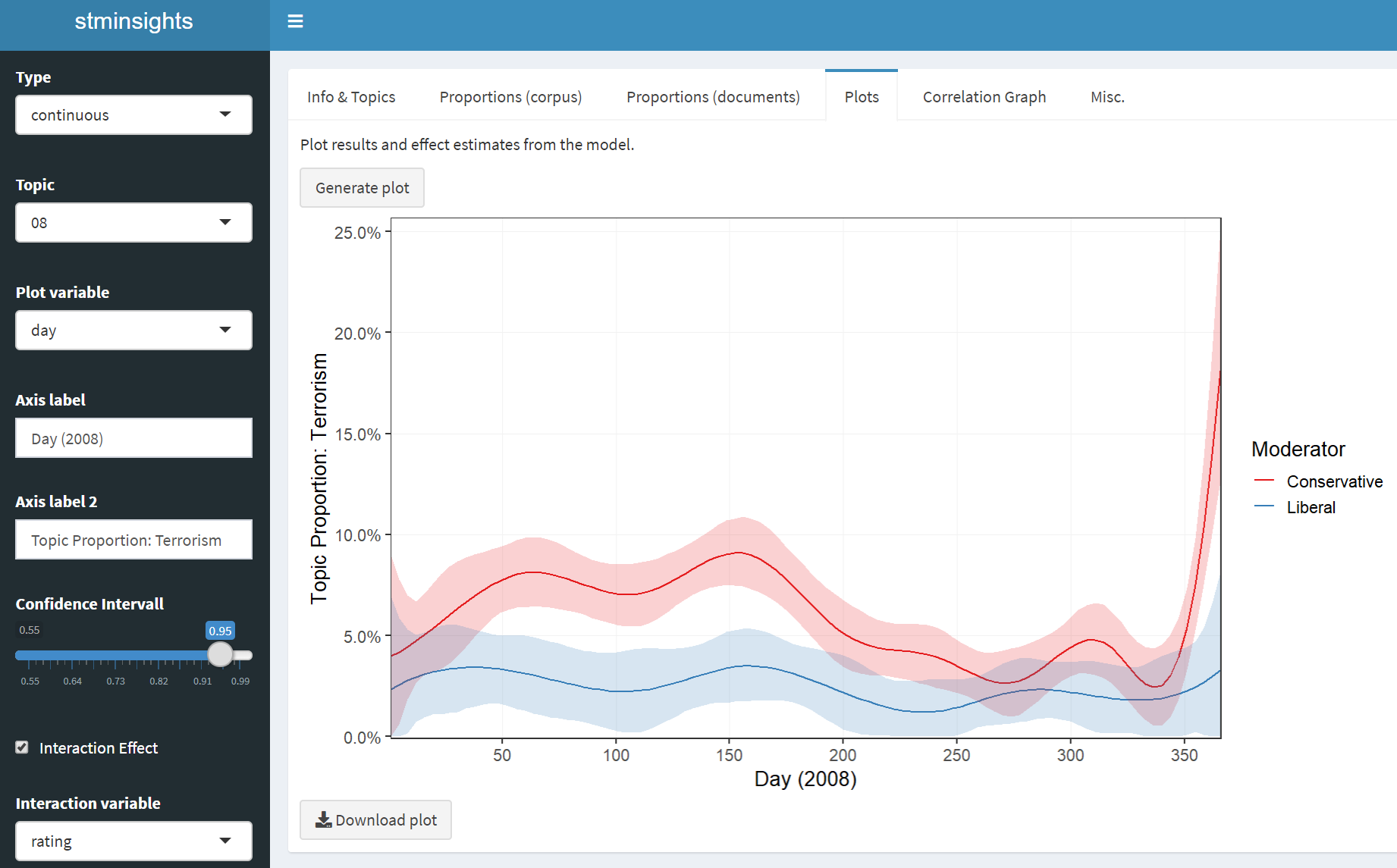## A Shiny Application for Inspecting Structural Topic Models

Topic models are widely used statistical models for reducing the dimensionality of textual data. Although the approach is quantitative in nature, model selection and validation of topic model results can be quite labor intensive, as it requires qualitative inspection of many documents and terms. This is were stminsights comes in: the package enables interactive validation, interpretation and visualization of one or several Structural Topic Models (stm). In case you are not familiar with structural topic models, the stm package vignette is an excellent starting point.

## How to Install

Stminsights can be installed from CRAN by running install.packages('stminsights').

You can also download the latest development version of the app by running devtools::install_github('cschwem2er/stminsights').

For Windows users installing from github requires proper setup of Rtools.

## How to Use

### Preparation

The main part of stminsights is an interactive shiny application, which requires a .RData file as input. This file should include:

• one or several stm objects.
• one or several estimateEffect objects.
• an object out which was used to fit your stm models and contains documents, vocabulary and metadata.

As an example, the following code uses the quanteda package to prepare the gadarian corpus for structural topic modeling. Afterwards, two models and estimates effects are computed and all objects required for stminsights are stored in stm_gadarian.RData:

library(stm)
library(quanteda)

# prepare data
data <- corpus(gadarian, text_field = 'open.ended.response')
docvars(data)$text <- as.character(data) data <- dfm(data, stem = TRUE, remove = stopwords('english'), remove_punct = TRUE) %>% dfm_trim(min_count = 2) out <- convert(data, to = 'stm') # fit models and effect estimates gadarian_3 <- stm(documents = out$documents,
vocab = out$vocab, data = out$meta,
prevalence = ~ treatment + s(pid_rep),
K = 3, verbose = FALSE)
prep_3 <- estimateEffect(1:3 ~ treatment + s(pid_rep), gadarian_3,
meta = out$meta) gadarian_5 <- stm(documents = out$documents,
vocab = out$vocab, data = out$meta,
prevalence = ~ treatment + s(pid_rep),
K = 5, verbose = FALSE)
prep_5 <- estimateEffect(1:5 ~ treatment + s(pid_rep), gadarian_5,
meta = out\$meta)

# save objects in .RData file
save.image('stm_gadarian.RData')

### Interactive application

After preparing the .RData file, the shiny application can be launched with run_stminsights():

#library(stminsights)
#run_stminsights()Hovering over UI elements displays tooltips that assist users in navigating through the application. Stminsights is organized as a dashboard with multiple columns that serve different purposes:

• Info & Topics:

• load the .RData file, select models and as effect estimates
• inspect the most important terms and documents for topics
• assign labels to topics
• Proportions (corpus):

• show proportions for each topic
• export proportion plots
• Proportions (documents):

• show topic proportions for each document
• export document topic proportion plots
• visualize and inspect document topic proportions interactively
• Plots:

• create different visualizations for the output of the selected model
• export effect plots
• Correlation Graph:

• create visualizations of the correlation network between topics
• export correlation plots
• Misc:

• calculate diagnostics for evaluating all models in the .RData
• get_effects(): create a dataframe including prevalence effects for one stm model
• get_network(): create a tidygraph for a correlation network of stm topics
• get_diag(): create a dataframe including statistical diagnostics for several models